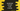# 3 different JavaScript program to convert a set to array## JavaScript program to convert a set to array:

Set holds unique values. We can’t have more than one same value in a JavaScript set. But in an array, we can have an item more than one time. Sometimes, we might need to convert a set to an array. In this post, I will show you how to convert a set to an array in JavaScript.

### Using Array.from():

Array.from is a static method that creates a new instance of an array with the passed iterable object. If we pass a set, it convers that set to an array.

``````let set = new Set();

let convertedArray = Array.from(set);
console.log(convertedArray);``````

It will print:

``[ 1, 2, 3, 4 ]``

Using spread syntax, we can convert a set to an array:

``````let set = new Set();

let convertedArray = [...set];
console.log(convertedArray);``````

It prints the same output.

### Using forEach:

We can always iterate throught the set items and insert them to an empty array. This is a not a recommended method to use.

``````let set = new Set();